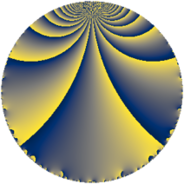# Properties

 Label 45.3.iLevel $45$ Weight $3$ Character orbit 45.i Rep. character $\chi_{45}(11,\cdot)$ Character field $\Q(\zeta_{6})$ Dimension $16$ Newform subspaces $1$ Sturm bound $18$ Trace bound $0$

# Related objects

## Defining parameters

 Level: $$N$$ $$=$$ $$45 = 3^{2} \cdot 5$$ Weight: $$k$$ $$=$$ $$3$$ Character orbit: $$[\chi]$$ $$=$$ 45.i (of order $$6$$ and degree $$2$$) Character conductor: $$\operatorname{cond}(\chi)$$ $$=$$ $$9$$ Character field: $$\Q(\zeta_{6})$$ Newform subspaces: $$1$$ Sturm bound: $$18$$ Trace bound: $$0$$

## Dimensions

The following table gives the dimensions of various subspaces of $$M_{3}(45, [\chi])$$.

Total New Old
Modular forms 28 16 12
Cusp forms 20 16 4
Eisenstein series 8 0 8

## Trace form

 $$16 q + 4 q^{3} + 16 q^{4} - 22 q^{6} + 2 q^{7} + 8 q^{9} + O(q^{10})$$ $$16 q + 4 q^{3} + 16 q^{4} - 22 q^{6} + 2 q^{7} + 8 q^{9} - 18 q^{11} - 22 q^{12} - 10 q^{13} - 54 q^{14} + 10 q^{15} - 32 q^{16} - 8 q^{18} - 52 q^{19} + 72 q^{21} - 24 q^{22} - 54 q^{23} + 108 q^{24} + 40 q^{25} + 34 q^{27} + 32 q^{28} - 54 q^{29} - 100 q^{30} + 32 q^{31} + 216 q^{32} + 62 q^{33} + 54 q^{34} - 86 q^{36} + 44 q^{37} + 252 q^{38} + 160 q^{39} - 30 q^{40} + 144 q^{41} - 270 q^{42} - 124 q^{43} + 140 q^{45} - 108 q^{46} - 216 q^{47} - 172 q^{48} - 54 q^{49} - 106 q^{51} + 62 q^{52} - 316 q^{54} - 18 q^{56} - 236 q^{57} + 90 q^{58} - 486 q^{59} - 10 q^{60} + 62 q^{61} - 132 q^{63} + 256 q^{64} - 90 q^{65} + 208 q^{66} + 14 q^{67} - 288 q^{68} + 90 q^{69} - 60 q^{70} + 804 q^{72} - 268 q^{73} + 540 q^{74} - 20 q^{75} - 106 q^{76} + 702 q^{77} + 290 q^{78} - 40 q^{79} - 112 q^{81} - 204 q^{82} + 522 q^{83} + 714 q^{84} + 30 q^{85} + 54 q^{86} + 106 q^{87} + 144 q^{88} + 250 q^{90} + 136 q^{91} - 1332 q^{92} + 90 q^{93} - 150 q^{94} + 180 q^{95} + 166 q^{96} - 142 q^{97} - 824 q^{99} + O(q^{100})$$

## Decomposition of $$S_{3}^{\mathrm{new}}(45, [\chi])$$ into newform subspaces

Label Dim $A$ Field CM Traces $q$-expansion
$a_{2}$ $a_{3}$ $a_{5}$ $a_{7}$
45.3.i.a $16$ $1.226$ $$\mathbb{Q}[x]/(x^{16} + \cdots)$$ None $$0$$ $$4$$ $$0$$ $$2$$ $$q+\beta _{3}q^{2}+(-\beta _{7}+\beta _{11})q^{3}+(2-\beta _{1}+\cdots)q^{4}+\cdots$$

## Decomposition of $$S_{3}^{\mathrm{old}}(45, [\chi])$$ into lower level spaces

$$S_{3}^{\mathrm{old}}(45, [\chi]) \cong$$ $$S_{3}^{\mathrm{new}}(9, [\chi])$$$$^{\oplus 2}$$